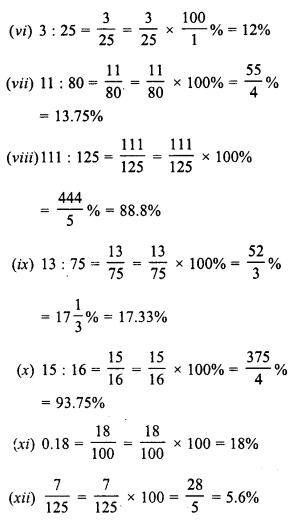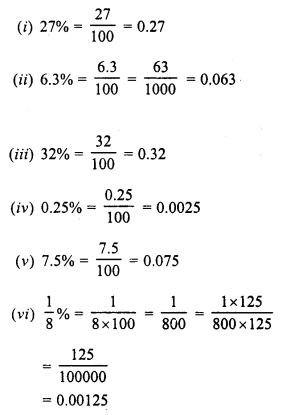## RD Sharma Class 8 Solutions Chapter 12 Percentage Ex 12.1

These Solutions are part of RD Sharma Class 8 Solutions. Here we have given RD Sharma Class 8 Solutions Chapter 12 Percentage Ex 12.1

Other Exercises

Question 1.
Write each of the following as percent: Solution—
(i) $$\frac { 7 }{ 25 }$$
(ii) $$\frac { 16 }{ 625 }$$
(iii) $$\frac { 5 }{ 8 }$$
(iv) 0.8
(v) 0.005
(vi) 3 : 25
(vii) 11 : 80
(viii) 111 : 125
(ix) 13 : 75
(x) 15 : 16
(xi) 0.18
(xii) $$\frac { 7 }{ 125 }$$
Solution:Question 2.
Convert the following percentages to fractions and ratios :
(i) 25%
(ii) 2.5%
(iii) 0.25%
(iv) 0.3%
(v) 125%
Solution:Question 3.
Express the following as decimal fractions :
(i) 27%
(ii) 6.3%
(iii) 32%
(iv) 0.25%
(v) 7.5%
(vi) $$\frac { 1 }{ 8 }$$ %
Solution:Hope given RD Sharma Class 8 Solutions Chapter 12 Percentage Ex 12.1 are helpful to complete your math homework.

If you have any doubts, please comment below. Learn Insta try to provide online math tutoring for you.